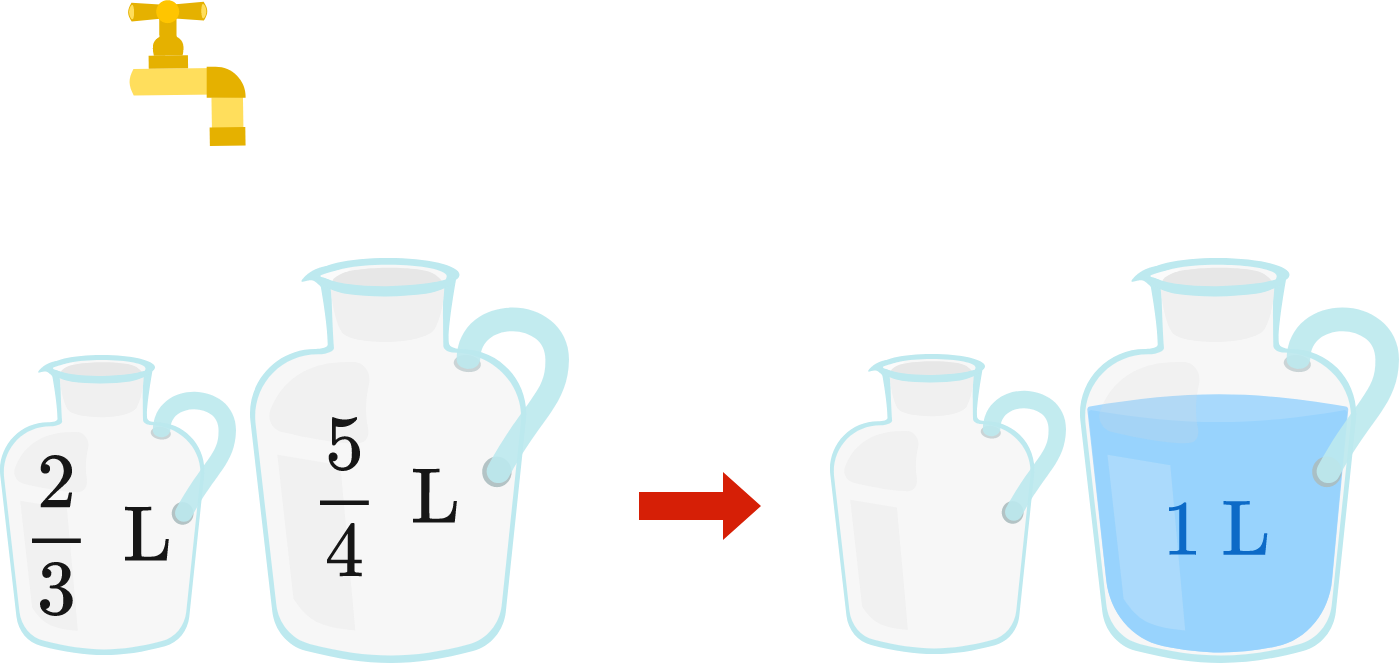# Jugs Riddle

Logic Level 3

I have 2 unmarked empty jugs of capacities $\frac{2}{3}$ liters and $\frac{5}{4}$ liters, as shown below left.

Because they are unmarked, I can only pour water from one jug to another until the initial jug is empty or the other is full. The unwanted water will be wasted into a sink, for there are no other containers.

After some pouring/filling/wasting, I measure exactly 1 liter of water in the bigger jug with the other empty, as shown.

Using the minimum amount of water to obtain the 1 liter, let $x$ be the number of times an empty jug is filled from the faucet, and let $y$ be the number of times a full jug is emptied into the sink. What is the value of $x+y?$×